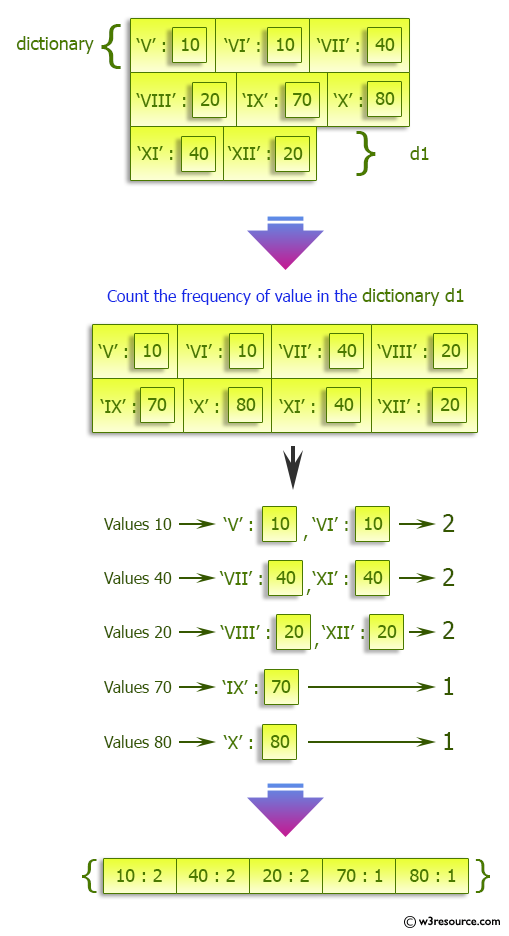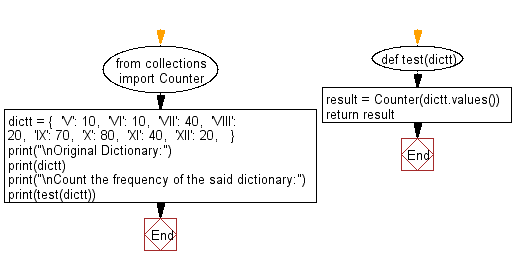﻿ Python: Count the frequency in a given dictionary - w3resource# Python: Count the frequency in a given dictionary

## Python dictionary: Exercise-61 with Solution

Write a Python program to count the frequency in a given dictionary.

Sample Solution:

Python Code:

``````from collections import Counter
def test(dictt):
result = Counter(dictt.values())
return result

dictt = {
'V': 10,
'VI': 10,
'VII': 40,
'VIII': 20,
'IX': 70,
'X': 80,
'XI': 40,
'XII': 20,
}

print("\nOriginal Dictionary:")
print(dictt)
print("\nCount the frequency of the said dictionary:")
print(test(dictt))
```
```

Sample Output:

```Original Dictionary:
{'V': 10, 'VI': 10, 'VII': 40, 'VIII': 20, 'IX': 70, 'X': 80, 'XI': 40, 'XII': 20}

Count the frequency of the said dictionary:
Counter({10: 2, 40: 2, 20: 2, 70: 1, 80: 1})
```

Pictorial Presentation:Flowchart:## Visualize Python code execution:

The following tool visualize what the computer is doing step-by-step as it executes the said program:

Python Code Editor:

Have another way to solve this solution? Contribute your code (and comments) through Disqus.

What is the difficulty level of this exercise?

Test your Programming skills with w3resource's quiz.

﻿

## Python: Tips of the Day

Clamps num within the inclusive range specified by the boundary values x and y:

Example:

```def tips_clamp_num(num,x,y):
return max(min(num, max(x, y)), min(x, y))
print(tips_clamp_num(2, 4, 6))
print(tips_clamp_num(1, -1, -6))
```

Output:

```4
-1
```